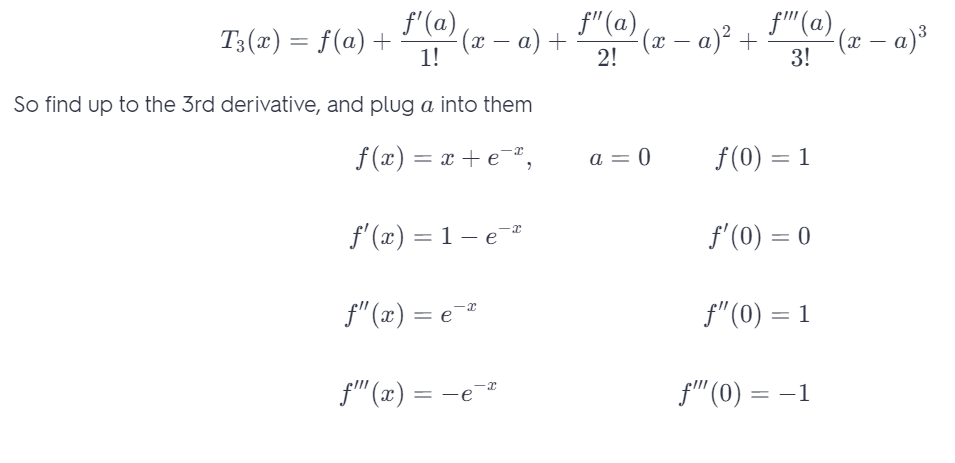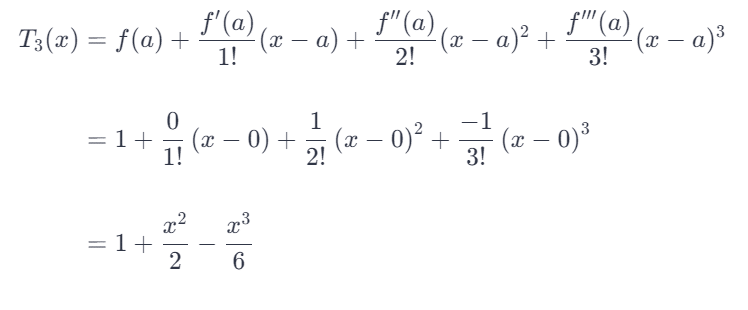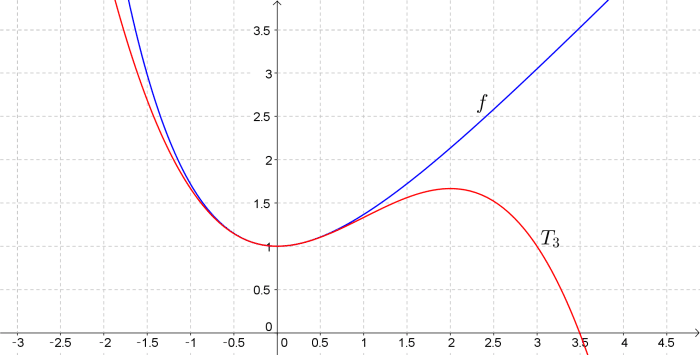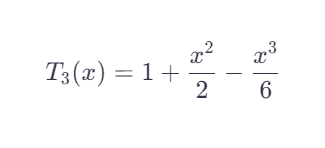# Find The Taylor Polynomial T3(X) For The Function F Centered At The Number A. F(X) = X + E−x, A = 0

We thoroughly check each answer to a question to provide you with the most correct answers. Found a mistake? Let us know about it through the REPORT button at the bottom of the page.

Find the Taylor polynomial T3(x) for the function f centered at the number a. Graph f and T3 on the same screen.
f(x) = x + e-^x, a=0

For T3 it isNow plug everything in